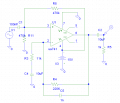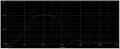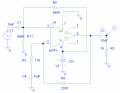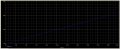# Help needed with amplifier in PSPICE

#### d_695

Joined Feb 27, 2011
5
I need help with analyzing this circuit using PSPICE. This is for a homework project. The specifications for this are as follows: I am to use a single ua741 opamp to design an amplifier with a gain of 20 volts, from a single negative power supply. It is to deliver 2 volts peak to a 1K load at 1 KHz. Input resistance must be greater than 100K and the frequency response needs to be from 50 Hz to 2 KHz +0/-3 dB (the low and high frequency cutoffs).

It looks like the circuit I have here does indeed have a gain of 20, but I need to figure out a few basic things, as I barely know how this amplifier works. I'm assuming that R5, within the high-pass filter at the output, is the 1K load here. I need to figure out how to measure for the appropriate frequency response.

This is the circuit in PSPICE:This is the graph of voltage versus frequency, showing a gain of at least 20 V between 56.971 Hz and 213.952 Hz.Now, when the specifications say 2 volts peak across a 1K load at 1 KHz, am I supposed to make the graph above reflect that (it's currently 12.210 V at 1 KHz), or am I thinking of something else? How can I tell if this design meets the frequency response requirements?

Thanks in advance for any help!

#### jut

Joined Aug 25, 2007
224
...the frequency response needs to be from 50 Hz to 2 KHz +0/-3 dB (the low and high frequency cutoffs).
I'm pretty sure this means at 50Hz, the gain should be +0dB. At 2k, the gain should be -3dB.

You know this formula, right? Gain in dB = 20*log10 (Voltage Gain)
So for you amp, Gain in dB = 20*log10 (20) = 26.02 dB

So another statement of you spec would go as follows. At 50 Hz the gain should be 26.02dB + 0dB. At 2kHz the gain should be no less than 26.02dB - 3dB = 23.02dB.

Let's look at this another way. Consider the above formula for gain in dB, but rearranged: 10^((Gain in dB)/20)=Voltage Gain

At 50Hz: 10^((26.02)/20)=Voltage Gain=20
At 2kHz: 10^((23.02)/20)=Voltage Gain=14.16

So another way of considering your specs: The voltage gain at 50Hz should be 20, the voltage gain at 2kHz should be no less than 14.16.

Your plot is not showing enough tick marks to assess your specs.

#### d_695

Joined Feb 27, 2011
5
So another way of considering your specs: The voltage gain at 50Hz should be 20, the voltage gain at 2kHz should be no less than 14.16.
Well, I found that if I remove capacitor C5 from what was the low-pass filter, and make a couple other adjustments like this:I get an output like this:(I don't think the squares on the line are tick marks, but rather just an indication of what the line is, in case the chart is printed.)

So at 50 Hz, the gain is 21, and at 2 KHz, the gain is also 21. I don't know what else I can do in terms of adding components to get this circuit to meet the specifications.

Also, how can I tell if this is delivering the 2-volts peak to the 1K load at 1 KHz? Is there a way I can measure for that in PSPICE?

Thanks again!

Joined Dec 26, 2010
2,148
Your present circuit would be expected to give a nominal gain of approximately (1+220/11) or 21.

If you want a gain of 20, you could obtain this by choosing resistors for the feedback network to give a ratio of 19:1 instead of 20:1, but this might require awkward values. Are you allowed to add an extra resistor to the feedback network?

#### jut

Joined Aug 25, 2007
224
>>Also, how can I tell if this is delivering the 2-volts peak to the 1K load at 1 KHz? Is there a way I can measure for that in PSPICE?

2 Vp into a 1k load (resistive) implies 2mA of current. I think the goal of that spec is to ensure the opamp can provide enough current. So, you can plot the current in the 1k load and make sure it hits 2mA when the voltage is 2Vp.

#### Ron H

Joined Apr 14, 2005
7,014
>>Also, how can I tell if this is delivering the 2-volts peak to the 1K load at 1 KHz? Is there a way I can measure for that in PSPICE?

2 Vp into a 1k load (resistive) implies 2mA of current. I think the goal of that spec is to ensure the opamp can provide enough current. So, you can plot the current in the 1k load and make sure it hits 2mA when the voltage is 2Vp.
Ohms law makes that a pretty sure thing.#### jut

Joined Aug 25, 2007
224
Ohms law makes that a pretty sure thing.Hehe, ya. That's what I was getting at in a round about way. I think the intent of the spec is to get the designer to think about the opamp's current supplying capability. In this case, the opamp should have no problem supplying 2mA. But if the load resistor were 100 ohm, the opamp's output no longer obeys ohm's law since it can't provide enough current needed to put 2V across a 100ohm resistor.

#### d_695

Joined Feb 27, 2011
5
>>Also, how can I tell if this is delivering the 2-volts peak to the 1K load at 1 KHz? Is there a way I can measure for that in PSPICE?

2 Vp into a 1k load (resistive) implies 2mA of current. I think the goal of that spec is to ensure the opamp can provide enough current. So, you can plot the current in the 1k load and make sure it hits 2mA when the voltage is 2Vp.
Like this?The voltage across R5 is about equal to the current in milliamps through R5 for any given value between 1 V and 21 V.

#### d_695

Joined Feb 27, 2011
5
Your present circuit would be expected to give a nominal gain of approximately (1+220/11) or 21.

If you want a gain of 20, you could obtain this by choosing resistors for the feedback network to give a ratio of 19:1 instead of 20:1, but this might require awkward values. Are you allowed to add an extra resistor to the feedback network?
I believe that there is no limit on the number of resistors I can use. The gain is indeed 21, but I found that's what I need in order to get a gain of 20 V at 50 Hz.

#### Ron H

Joined Apr 14, 2005
7,014
I believe that there is no limit on the number of resistors I can use. The gain is indeed 21, but I found that's what I need in order to get a gain of 20 V at 50 Hz.
Your first post led me to believe that the -3dB corners were to be at 50Hz and 2kHz, and the midband gain was to be 20. If this is the case, then you should set the gain at around 300Hz, where it is flat.